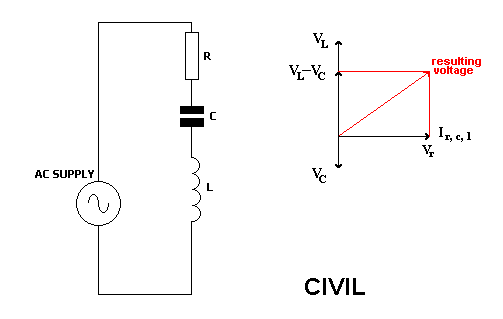Home > Electronic Tutorials > Phasors and Resonance Tutorial > L, C and R in Series in AC Circuits Tutorial

Electronic Tutorials - Phasors and Resonance

# L, C and R in Series in AC Circuits TutorialCIVIL, This means that for  C, I leads V.

Since all the components are in series, the same current flows through them all, as indicated by the horizontal phasor.

Resistor voltage and current are in phase, so resistor voltage is shown also along this phasor.

Inductor voltage leads the current by 90 degrees.

Capacitor voltage lags the current by 90 degrees.

TO FIND THE RESULTING VOLTAGE

Since the voltages across C and L are 180 degrees out of phase, then the result of these two is one subtracted from the other.

Inductor voltage minus capacitor voltage.

This result can be combined with the resistor voltage, by using Pythagoras.

The final result is the applied voltage across the circuit.